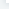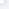# NumPy 统计函数

NumPy 统计函数NumPy 提供了很多统计函数，用于从数组中查找最小元素，最大元素，百分位标准差和方差等。 函数说明如下：numpy.amin() 和 numpy.amax()numpy.amin() 用于计算数组中的元素沿指定轴的最小值。numpy.amax() 用于计算数组中的元素沿指定轴的最大值。实例import numpy as npa = np.array([[3,7,5],[8,4,3],[2,4,9]])print ('我们的数组是：')print (a)print ('\n')print ('调用 amin() 函数：')print (np.amin(a,1))print ('\n')print ('再次调用 amin() 函数：')print (np.amin(a,0))print ('\n')print ('调用 amax() 函数：')print (np.amax(a))print ('\n')print ('再次调用 amax() 函数：')print (np.amax(a, axis = 0))输出结果为：我们的数组是：[[3 7 5] [8 4 3] [2 4 9]]调用 amin() 函数：[3 3 2]再次调用 amin() 函数：[2 4 3]调用 amax() 函数：9再次调用 amax() 函数：[8 7 9]numpy.ptp()numpy.ptp()函数计算数组中元素最大值与最小值的差（最大值 - 最小值）。实例import numpy as npa = np.array([[3,7,5],[8,4,3],[2,4,9]])print ('我们的数组是：')print (a)print ('\n')print ('调用 ptp() 函数：')print (np.ptp(a))print ('\n')print ('沿轴 1 调用 ptp() 函数：')print (np.ptp(a, axis = 1))print ('\n')print ('沿轴 0 调用 ptp() 函数：')print (np.ptp(a, axis = 0))输出结果为：我们的数组是：[[3 7 5] [8 4 3] [2 4 9]]调用 ptp() 函数：7沿轴 1 调用 ptp() 函数：[4 5 7]沿轴 0 调用 ptp() 函数：[6 3 6]numpy.percentile()百分位数是统计中使用的度量，表示小于这个值的观察值的百分比。 函数numpy.percentile()接受以下参数。numpy.percentile(a, q, axis)参数说明：a:

# 50% 的分位数，就是 a 里排序之后的中位数print (np.percentile(a, 50))

# axis 为 0，在纵列上求print (np.percentile(a, 50, axis=0))

# axis 为 1，在横行上求print (np.percentile(a, 50, axis=1))

# 保持维度不变print (np.percentile(a, 50, axis=1, keepdims=True))输出结果为：我们的数组是：[[10 7 4] [ 3 2 1]]调用 percentile() 函数：3.5[6.5 4.5 2.5][7. 2.][[7.] [2.]]numpy.median()numpy.median() 函数用于计算数组 a 中元素的中位数（中值）实例import numpy as npa = np.array([[30,65,70],[80,95,10],[50,90,60]])print ('我们的数组是：')print (a)print ('\n')print ('调用 median() 函数：')print (np.median(a))print ('\n')print ('沿轴 0 调用 median() 函数：')print (np.median(a, axis = 0))print ('\n')print ('沿轴 1 调用 median() 函数：')print (np.median(a, axis = 1))输出结果为：我们的数组是：[[30 65 70] [80 95 10] [50 90 60]]调用 median() 函数：65.0沿轴 0 调用 median() 函数：[50. 90. 60.]沿轴 1 调用 median() 函数：[65. 80. 60.]numpy.mean()numpy.mean() 函数返回数组中元素的算术平均值。 如果提供了轴，则沿其计算。算术平均值是沿轴的元素的总和除以元素的数量。实例import numpy as npa = np.array([[1,2,3],[3,4,5],[4,5,6]])print ('我们的数组是：')print (a)print ('\n')print ('调用 mean() 函数：')print (np.mean(a))print ('\n')print ('沿轴 0 调用 mean() 函数：')print (np.mean(a, axis = 0))print ('\n')print ('沿轴 1 调用 mean() 函数：')print (np.mean(a, axis = 1))输出结果为：我们的数组是：[[1 2 3] [3 4 5] [4 5 6]]调用 mean() 函数：3.6666666666666665沿轴 0 调用 mean() 函数：[2.66666667 3.66666667 4.66666667]沿轴 1 调用 mean() 函数：[2. 4. 5.]numpy.average()numpy.average() 函数根据在另一个数组中给出的各自的权重计算数组中元素的加权平均值。该函数可以接受一个轴参数。 如果没有指定轴，则数组会被展开。 加权平均值即将各数值乘以相应的权数，然后加总求和得到总体值，再除以总的单位数。考虑数组[1,2,3,4]和相应的权重[4,3,2,1]，通过将相应元素的乘积相加，并将和除以权重的和，来计算加权平均值。加权平均值 = (1*4+2*3+3*2+4*1)/(4+3+2+1)实例import numpy as npa = np.array([1,2,3,4])print ('我们的数组是：')print (a)print ('\n')print ('调用 average() 函数：')print (np.average(a))print ('\n')

# 不指定权重时相当于 mean 函数wts = np.array([4,3,2,1])print ('再次调用 average() 函数：')print (np.average(a,weights = wts))print ('\n')

# 如果 returned 参数设为 true，则返回权重的和 print ('权重的和：')print (np.average([1,2,3, 4],weights = [4,3,2,1], returned = True))输出结果为：我们的数组是：[1 2 3 4]调用 average() 函数：2.5再次调用 average() 函数：2.0权重的和：(2.0, 10.0)在多维数组中，可以指定用于计算的轴。实例import numpy as npa = np.arange(6).reshape(3,2)print ('我们的数组是：')print (a)print ('\n')print ('修改后的数组：')wt = np.array([3,5])print (np.average(a, axis = 1, weights = wt))print ('\n')print ('修改后的数组：')print (np.average(a, axis = 1, weights = wt, returned = True))输出结果为：我们的数组是：[[0 1] [2 3] [4 5]]修改后的数组：[0.625 2.625 4.625]修改后的数组：(array([0.625, 2.625, 4.625]), array([8., 8., 8.]))标准差标准差是一组数据平均值分散程度的一种度量。标准差是方差的算术平方根。标准差公式如下：std = sqrt(mean((x - x.mean())**2))如果数组是 [1，2，3，4]，则其平均值为 2.5。 因此，差的平方是 [2.25,0.25,0.25,2.25]，并且其平均值的平方根除以 4，即 sqrt(5/4) ，结果为 1.1180339887498949。实例import numpy as npprint (np.std([1,2,3,4]))输出结果为：1.1180339887498949方差统计中的方差（样本方差）是每个样本值与全体样本值的平均数之差的平方值的平均数，即 mean((x - x.mean())** 2)。 换句话说，标准差是方差的平方根。实例import numpy as npprint (np.var([1,2,3,4]))输出结果为：1.25

NumPy 统计函数

## 相关文章